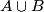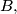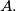### IMO Shortlist 1996 problem C4

Kvaliteta:
Avg: 0,0
Težina:
Avg: 7,0
Determine whether or nor there exist two disjoint infinite sets$A$ and$B$ of points in the plane satisfying the following conditions:

a.) No three points in$A \cup B$ are collinear, and the distance between any two points in$A \cup B$ is at least 1.

b.) There is a point of$A$ in any triangle whose vertices are in$B,$ and there is a point of$B$ in any triangle whose vertices are in$A.$
Izvor: Međunarodna matematička olimpijada, shortlist 1996